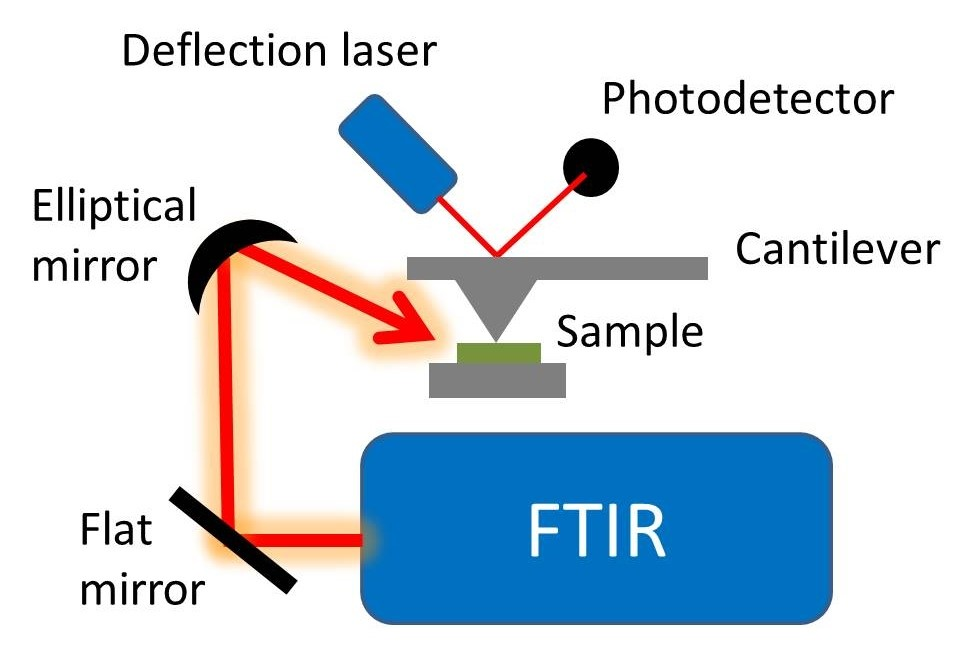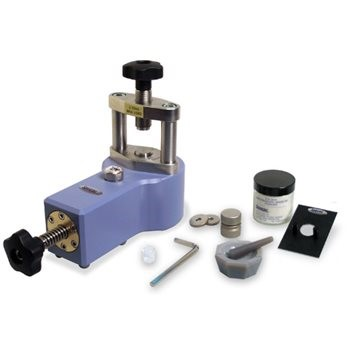# What is Fourier Transform Infra Red Spectroscopy, Introduction ,Principle, Advantages,Factors ?

0

### Introduction:

Fourier Transform Infra Red Spectroscopy is abbreviated as FTIR spectroscopy. It is a technique used to observe the infra red spectrum of absorption of unknown compound. From the absorption it is used to identifies the chemical bond in the molecule.

READ  Electrochemistry Introduction, Principle, Conductivity, Factors affecting electrolytic conductance,Debye – Huckel and Onsager equation, Kohlraush’s law, Nernst Equation etc..?In this technique, the sample is made into a pellet with Potassium bromide which is taken as standard in the hydraulic press machine. And then it is placed under the infra red radiation it absorbs the light and gives absorption bands at various frequency. From that transmitted signal we can predict the unknown compound.

READ  Copper sulphate Formula, Structure, Uses and Video### Principle:

FTIR also follows the same principle as in the case of IR spectroscopy which means the molecule that is having change in dipole moment only undergoes this kind of spectrum and records the signals.

READ  What is Sodium Carbonate, Balancing equation , uses and Videos etc...

But in FTIR, the measurement was done over broad range of spectrum instead of narrow range of spectrum as in the case of IR spectroscopy.

### Range:

FTIR is used to measure the light absorption of wave number from 4000 to 400 cm-1.

READ  What is Raman Spectroscopy, Introduction, Principle, Types of scattering, Applications ?

### Difference between IR and FTIR spectroscopy:

• The signal to noise ratio is lower in the case of IR, but in the case of FTIR that ratio is preferentially higher.
• In FTIR, the accuracy of wave number is high.

### Advantage of FTIR over IR spectroscopy:

• High data collection speed
• Easy maintenance and speed
• High reproducibility of data
• Great spectral quality

### Factors affecting FTIR spectroscopy:

1. Bond order:

The number of bonds within the molecule decides the absorption bands of FTIR.

1. Inductive effect and mesomeric effect:
READ  First Law of Thermodynamics Introduction, Principle, Types of Systems, Properties, Zeroth law of Thermodynamics ?

The presence of inductive effect and mesomeric effect strengthens the bond which in turn changes the signals in the spectrum.

• Hydrogen bonding:

The presence of intramolecular and intermolecular hydrogen bonds also strengthens the molecule which affects the expected spectrum.